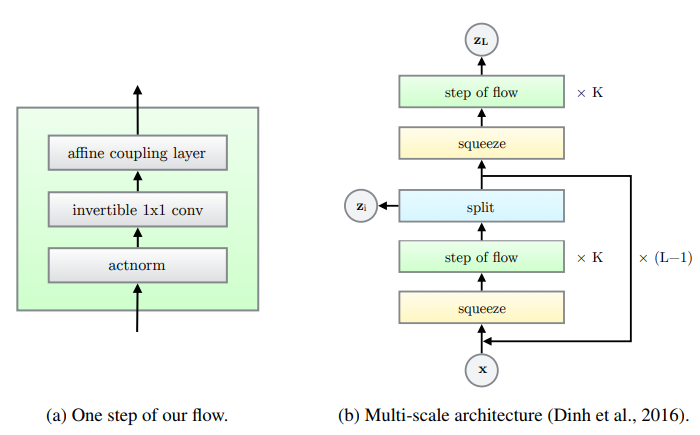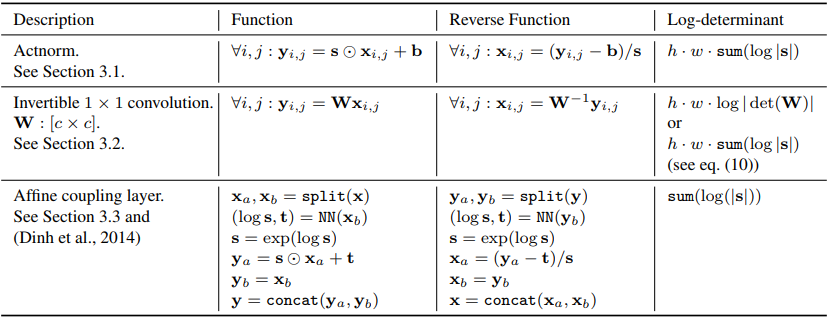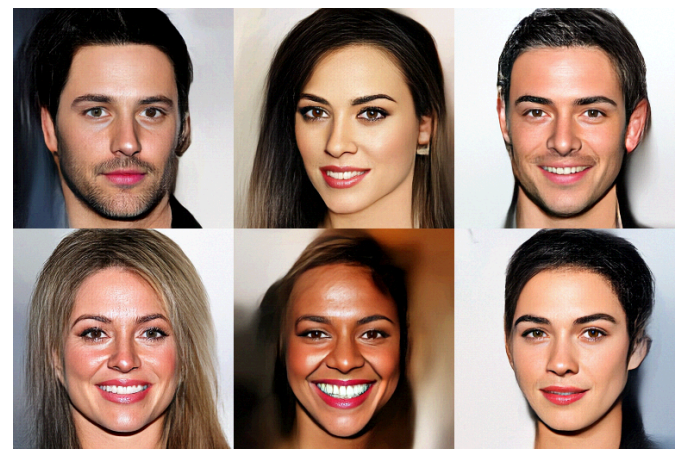# Glow: Generative Flow with Invertible 1x1 Convolutions

## WHY?

The motivation is almost the same as that of NICE, and RealNVP.

## WHAT?

The architecture of generative flow(Glow) is almost the same as multi-scale architecture of RealNVP.A step of flow of Glow uses act-norm instead of batch-norm and uses invertible 1x1 convolution instead of reverse ordering. Act-norm performs channel-wise normalization as Instance Normalization (IN). Since channel-wise masking keep the half of the channels unchanged, permutation of input is need to ensure all the inputs are used. Instead of revsersing the order of channels, 1x1 convolution can be used as channel-wise permutation. However, getting the determinant of W(channel to channel: c x c) is not easy. Thus, we can initialize W by first sampling a random rotation matrix whose log-determinant is 0. Next, perform LU decomposition to W to get P, L(lower triangular matrix) and U(Upper triangular matrix). Then, getting the determinant of W becomes much easier.

W = PL(U + diag(s))\\
\log|\det(W)| = \sum(\log|s|)

Lastly, affine coupling layer is added to a step of flow. This step of flows is stacked to form a multi-scale architecture.## So?Glow showed better result in CIFAR10, ImageNet, LSUN, and CelebA than Real NVP.

## Critic

Big improvement on flow based model but quality of generation is still somewhat unnatural.

Powered by aiden